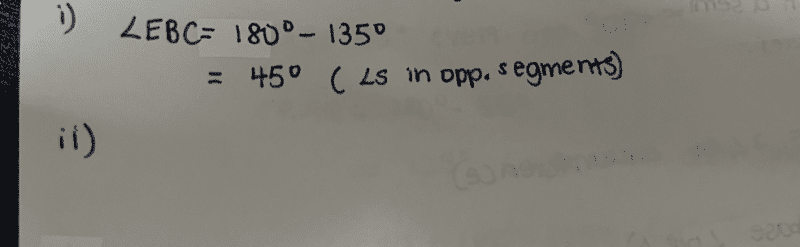# Question

Let me try.

Sum of interior angles in a polygon of n sides = (n-2) * 180
So, for an octagon, an interior angle = ((8-2)*180) / 8 = 135

(i) If we consider BCDE as a regular polygon,
then sum of its interior angles = 2*180 = 360

So angle EBC = (360 – 135 – 135) / 2 = 45

(ii) angle EBC = 135 – 45 = 90

(iii) angle DCE = (180 – 135) / 2 = 22.5